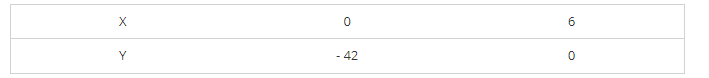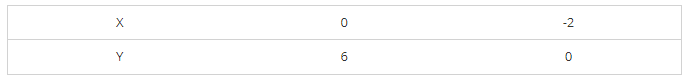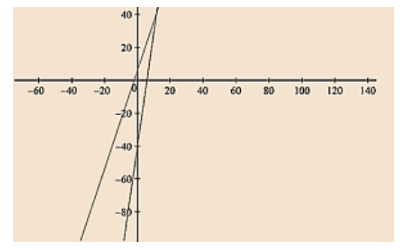# Ravish tells his daughter Aarushi, "Seven years ago, I was seven times as old as you were then.

Question:

Ravish tells his daughter Aarushi, "Seven years ago, I was seven times as old as you were then. Also, three years from now, I shall be three times as old as you will be". If present ages of Aarushi and Ravish are x and y years respectively, represent this situation algebraically as well as graphically.

Solution:

We are given the present age of Ravish as y years and Aarushi as x years.

Age of Ravish seven years ago = y - 7

Age of Aarushi seven years ago = x – 7

It has already been said by Ravish that seven years ago he was seven times old then Aarushi was then So, y - 7 = 7( x – 7)

y – 7 = 7x – 49

7x - y = - 7 + 49

7x – y – 42 = 0 .... (1)

Age of Ravish three years from now = y + 3

Age of Aarushi three years from now = x + 3

It has already been said by Ravish that three years from now he will be three times older then Aarushi will be then So, Y + 3 = 3 (x + 3)

y + 3 = 3x + 9

3x + 9 – y – 3 = 0

3x – y + 6 = 0 ..... (2)

(1) and (2) are the algebraic representation of the given statement.

We are given, 7x - y- 42 = 0

We get, y = 7x – 42

Now, substituting x = 0 in y = 7x – 42, we get y = – 42

Substituting x = 6 in y = 7x - 42, we get y = 0

Thus, we have the following table exhibiting the abscissa and ordinates of points on the line represented by the given equationWe are given, 3x – y + 6 = 0

We get, Y = 3x + 6

Now, substituting x = 0 in y = 3x + 6, We get y = 6

Substituting x = -2 in y = 3x + 6,

We get y = 0

Thus, we have the following table exhibiting the abscissa and ordinates of points on the line represented by the given equationThe red – line represents the equation 7x - y - 42 = 0.

The blue-line represents the equation 3x - y + 6 = 0.